# Maharashtra Board Class 5 Maths Solutions Chapter 15 Patterns Problem Set 53

Balbharti Maharashtra Board Class 5 Maths Solutions Chapter 15 Patterns Problem Set 53 Textbook Exercise Important Questions and Answers.

## Maharashtra State Board Class 5 Maths Solutions Chapter 15 Patterns Problem Set 53

Question 1.
Find the square numbers from the list given below.
5, 9, 12, 16, 50, 60, 64, 72, 80, 81
9,16, 64, 81, 4, 25, 49 are square numbers.Question 2.
Which are the triangular numbers in the given list?
3, 6, 8, 9, 12, 15, 16, 20, 21, 42
3, 6, 15, 21, 28, 10, 45, 55 are triangular numbers.

Question 3.
Name a number which is square as well as triangular.
36 is square as well as triangular number.

Question 4.
If 4 is the first square number, which is the tenth one?
121 is the tenth square number.Question 5.
If 3 is the first triangular number, which is the tenth one?
66 is the tenth triangular number.

• How will you decide if a given number is a square number?
• How will you decide if a given number is a triangular number?
• How many square numbers do you think there are?
• How many triangular numbers do you think there are?

Activity

Make a collection of pictures in which you can see square or triangular numbers.

Patterns in floor tiles

The tiles in each picture below form a specific pattern. Observe that there is no gap or open ground between two tiles.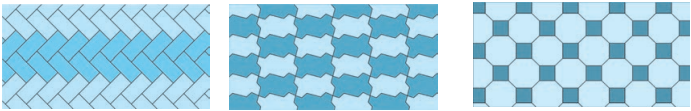On a large piece of card sheet, draw several shapes like the one shown alongside. Colour half of them. Cut them all out and separate them.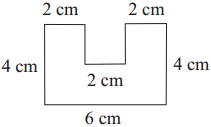One pattern made of these shapes is shown alongside. Make some other patterns of your own.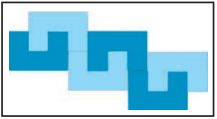Cut out many pieces of each of the shapes shown alongside. Join them in a pattern like floor tiles.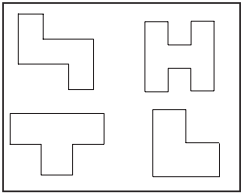Note the pattern and complete the design.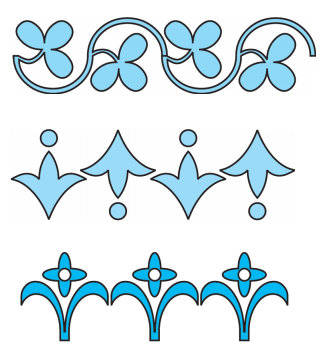Make your own shapes and use them to make patterns for sari and shawl borders, etc.

Perimeter and Area Problem Set 49 Additional Important Questions and Answers

Solve the following :

Question 1.
If 4 is the first square number which is the eighth one?
81 is the eighth square number.Question 2.
If 3 is the first triangular number which is the eighth one?
45 is the eighth triangular number.

Question 3.
Classify the following into square numbers and triangular numbers.
3, 4, 9,10,15,16; 45, 49, 64, 66, 81, 91
Square Numbers : 4, 9,16, 49, 64, 81
Triangular Numbers : 3, 10, 15, 45, 66, 91

Question 4.
Find out the numbers which are neither square nor triangular numbers from the following.
4, 5, 6, 8, 9, 10, 14, 15, 16, 25, 26, 27, 28.
5, 8 14, 26 and 27Question 5.
(1) If 4 is the first square number, which is the fifth one?
(2) If 3 is the first triangular number, which is the sixth one?
(3) Write all the square numbers between 20 and 80.
(4) Write all the triangular numbers between 20 and 80.
(5) Write the greatest two-digit square numbers as well as triangular numbers.
(6) Write the next three square numbers, 36, 49, 64,…….,
(7) Write the next three triangular numbers 36, 45, 55,
(1) 36
(2) 28
(3) 25, 36, 49, 64
(4) 21, 28, 36, 45, 55, 66, 78
(5) 81, 91
(6) 81, 100, 121
(7) 66, 78, 91Question 6.
Match the columns

 A B (1) Third square number (a) 15 (2) Fourth triangular number (b) 36 (3) Number neither square nor triangular (c) 16 (4) Number is both square as well as triangular number (d) 35